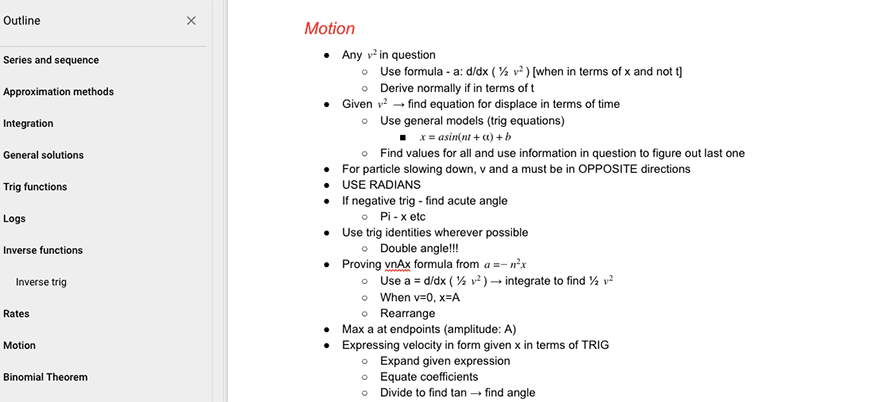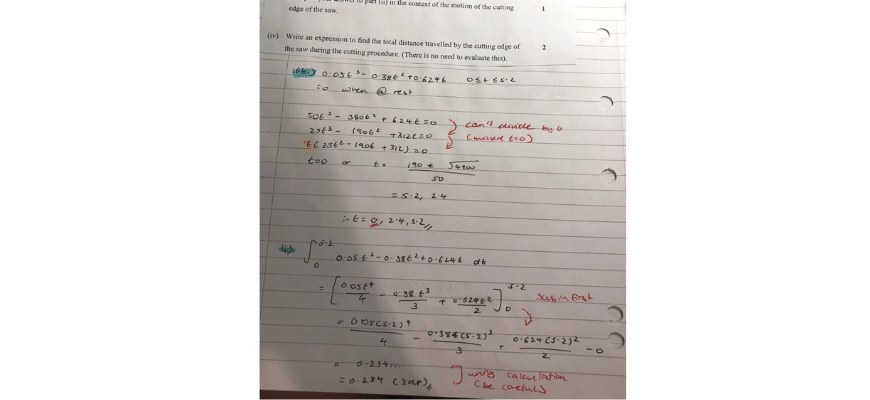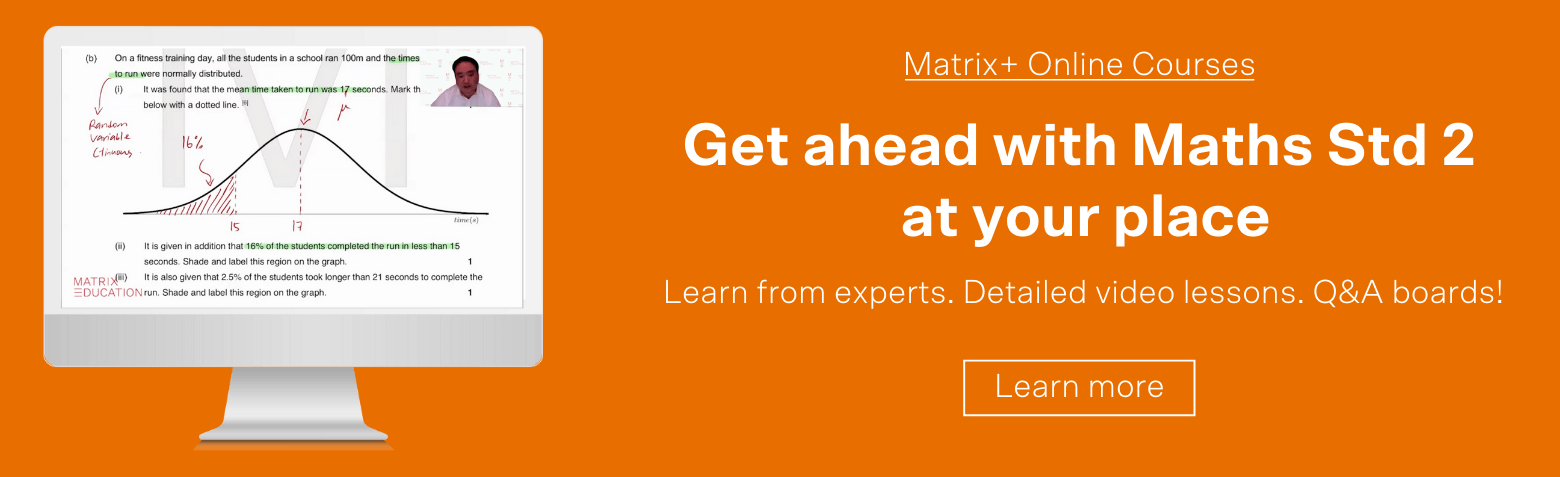# Beginner’s Guide to Year 12 Maths Standard 2 | Overview

Studying Maths Standard 2 doesn't have to be hard! In our Beginner's Guide, we will break down each topic to help you ace your HSC.Are you struggling with some Maths Standard 2 questions? Well, don’t worry! In our Beginner’s Guide to Year 12 Maths Standard 2, we will break down all of the NESA learning outcomes and provide you with some practice questions to help you tackle everything.

## All about the Beginner’s Guide to Year 12 Maths Standard 2

Now that you are in Year 12, it is essential that you build on your foundational knowledge from Years 7-11 and grow that knowledge further.

This Guide introduces through Year 12 Maths Standard 2 HSC content and skills that you need to master!

We will break down each concept into clear, concise and manageable chunks of theory, and provide you with some practice questions and worksheets.

Our purpose for writing this guide is to create clarity around the Year 12 Maths Standard 2 Syllabus.

## What’s in this guide?

### 1. Algebra

• Formulae & Equations
• Linear Relationships
• Simultaneous Equations
• Non-Linear Relationships

### 2. Trigonometry

• Review of Basic Trigonometry
• Area of a Triangle, Sine Rule, Cosine Rule
• Angle of Elevation & Depression

### 3. Scale Drawings & Maps

• Scale Drawings and Maps
• Simplifying a map scale
• Measurements from a map
• The trapezoidal rule to estimate area
• Rainfall over an area

### 4. Rates & Ratios

• Simplifying a ratio
• Converting ratios between units
• Dividing a quantity in a given ratio
• Map scales
• Converting between units of rates
• Using rates to make comparisons
• Energy problems with rates

## How will this Guide help me?

Our guides aim to clarify any confusion you might have with the syllabus topics!

We break down the topics and provide challenging questions at the end of each article to help you prepare for the HSC.

We also examine concepts behind different questions, instead of examining pure calculations. This is because we believe that it is beneficial for you to understand Maths, as opposed to simply listing steps to the right answer.

This is our philosophy behind our Matrix Maths Method.

## What are some common student learning difficulties?

In Year 12 Maths Std, many students come across these issues:

• A poor understanding of Algebra. All Maths topics require some basic understanding of Algebra. So, many students who have a poor understanding of Algebra will severely struggle with Year 12 Maths Std topics.

For example: Find the $$x$$ for the following quadratic equation:

$$y = x^{2} = 6x = 16$$

Students who struggle with this question may be comfortable with factorisation. However, they might be unfamiliar with other Mathematical methods like completing the square of the quadratic formula. These are necessary to solve the question.

• Inability to link concepts together. If students lack conceptual understanding of the topics in Maths Std 2, they will struggle to tackle questions which rely on multiple concepts.

For example: Find the turning point of the parabola $$y = x^{2} + 5mx + m$$.

To solve this question, students need to have a strong conceptual understanding of the link between the turning point, parabola, and quadratic inequalities.

• Struggle to communicate the topics properly. When students rote learn maths, they lack a strong conceptual understanding of the topics. This often causes them to struggle with communicating topics.
• Unable to do difficult questions. Many students come across a difficult question and give up without trying it. This is detrimental as it impedes their confidence in answering more challenging questions in the future.

## What do Band 6 students do differently?

So, now that we know what the most common Maths issues are, let’s examine how you can overcome these issues!

• Have a strong algebraic understanding. Don’t simply push through your Year 11 and 12 contents. Instead, Band 6 students have an awareness of their weaknesses, and consistently go back to topics from previous years and practise algebraic skills.

• Keep a logbook of difficult questions. Many students do practice questions to improve. However, when they reach a difficult question, they simply skip it! This is ineffective, because you are not expanding your skillset.  So, instead, you should keep a logbook of all the difficult questions and allocate some time to figure out the process.Image: Jessy’s logbook of difficult questions

• Consistently give yourself feedback. How can you improve if you don’t mark your own work and figure where you went wrong? So, you should keep a logbook of all the questions you answered incorrectly, then write the correct solution. You should revisit these questions before the exam to refresh your skills.• Be inquisitive. You should always ask questions if you don’t understand something, or want clarification! Remember, if you have a stronger understanding of a Mathematical concept, it will be easier to solve the questions.

• Be disciplined. Don’t just study for Maths the day before your exams! That’s not enough time. Maths is a subject that you need to consistently learn and practice. So, set some time to understand and break down a concept. Then make it your mission to continue doing practice questions to reinforce your knowledge.

• Communicate what you understand. The best way to learn is to teach someone else! So, explain your Maths concepts to your friends, and guide them through the steps of different Maths questions. This will help you remember and understand the concepts better, and it will also reveal areas that you don’t completely understand.

## Save time and boost your Maths Std 2 marks!

Our HSC experts will break down Mathematical concepts into manageable chunks through our structured video lessons. Learn more about Matrix+ Online Courses now.© Matrix Education and www.matrix.edu.au, 2021. Unauthorised use and/or duplication of this material without express and written permission from this site’s author and/or owner is strictly prohibited. Excerpts and links may be used, provided that full and clear credit is given to Matrix Education and www.matrix.edu.au with appropriate and specific direction to the original content.